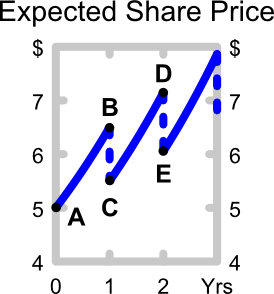# Fight Finance

#### CoursesTagsRandomAllRecentScores

The perpetuity with growth formula, also known as the dividend discount model (DDM) or Gordon growth model, is appropriate for valuing a company's shares. $P_0$ is the current share price, $C_1$ is next year's expected dividend, $r$ is the total required return and $g$ is the expected growth rate of the dividend.

$$P_0=\dfrac{C_1}{r-g}$$

The below graph shows the expected future price path of the company's shares. Which of the following statements about the graph is NOT correct?